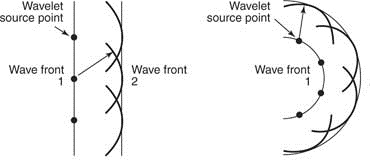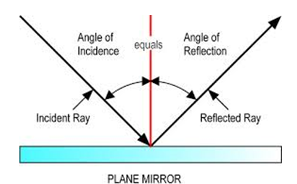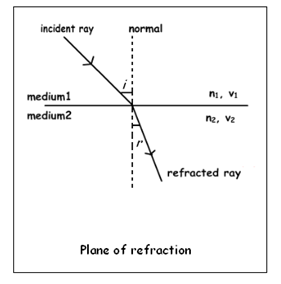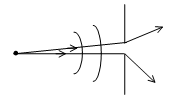#### Thank you for registering.

One of our academic counsellors will contact you within 1 working day.

Click to Chat

1800-1023-196

+91-120-4616500

CART 0

• 0
MY CART (5)

Use Coupon: CART20 and get 20% off on all online Study Material

ITEM
DETAILS
MRP
DISCOUNT
FINAL PRICE
Total Price: Rs.

There are no items in this cart.
Continue Shopping• Complete JEE Main/Advanced Course and Test Series
• Offered Price: Rs. 15,900
• View Details

# Revision Notes on Wave Optics

Huygens Principle:- Wave-front  of a wave, at any instant , is defined as the locus of all the particles in the medium which are being disturbed at the same instant of time and are in the same phase of vibration.

(a) Each point on a wave front acts as a source of new disturbance and emits its own set of spherical waves called secondary wavelets. The secondary wavelets travel in all directions with the velocity of light so long as they move in the same medium.(b) The envelope or the locus of these wavelets in the forward direction gives the position of new wave front at any subsequent time.

• Determination of Phase Difference:-

The phase difference between two waves at a point will depend upon

(a) The difference in path lengths of the two waves from their respective sources.

(b) The refractive index of the medium

(c) Initial phase difference between the source if any.

(d) Reflections, if any, in the path followed  by waves.

• Reflection of plane wave at plane surface (Laws of reflection):-

?(a) The incident ray, the reflected ray and normal to the reflecting surface at the point of incidence, all lie in one plane and that plane is perpendicular to the reflecting surface.

(b) The angle of incidence is equal to the angle of reflection.

So, ∠i = ∠r

This signifies angle of incidence is equal to the angle of reflection.

• Refraction of light:-

Refraction is the phenomena by virtue of which a wave going from one medium to another undergoes a change in velocity.(a) The sine of the angle between the incident ray and the normal bears a constant ratio to the sine of the angle between refracted ray and the normal.

sin i/sin r = v1/v2 = 1µ2 = constant

Here, v1 and v2 are the velocities of sound in first and second medium respectively.1µ2 is the refractive index of the second medium with respect to first.

(b) The incident ray, the refracted ray and the normal to the refracting surface lie in the same plane.

• Interference:- The modification in the distribution of light energy obtained by the superposition of two or more waves is called interference.

• Principle of superposition:- It states that a number of waves travelling, simultaneously, in a medium behave independent of each other and the net displacement of the particle, at any instant, is equal to the sum of the individual displacements due to all the waves.

• Displacement equation:- y R sin 2π/λ (vt+x/2)

• Amplitude:- R = 2a cos πx/λ

• Intensity:- I = K4a2 cos2 (πx/λ)    [I = KR2]

• Maxima:- A point having maximum intensity is called maxima.

x = 2n (λ/2)

A point will be a maxima if the two waves reaching there have a path difference of even multiple of λ/2.

Imax = 4Ka2 = 4i   (Here, i = Ka2)

• Minima:- A point having minimum intensity is called a minima.

x = (2n+1) (λ/2)

A point will be a minima if the two waves reaching there have a path difference of odd multiple of λ/2.

Imin = K. 4a2×0 = 0

• Condition for constructive interference:-

Path difference = (2n)λ/2

Phase difference = (2n)π

• Condition for destructive interference:-

Path difference = (2n+1)λ/2

Phase difference = (2n+1)π

• Coherent Sources:- Coherent sources are the sources  which either have no phase difference or have a constant difference of phase between them.
• Conditions for interference:-

(a) The two sources should emit, continuously, waves of same wavelength or frequency.

(b) The amplitudes of the two waves should be either or nearly equal

(c) The two sources should be narrow.

(d) The sources should be close to each other.

(e) The two sources should be coherent one.

• Young’s double slit experiment:-

Path difference, x = yd/D

Maxima, y = nλD/d

Here, n = 0,1,2,3….

Minima, y = (2n+1) λD/d

Here, n = 0,1,2,3….

• Fringe Width:- It is the distance between two consecutive bright and dark fringes.

β = λD/d

• Displacement of fringes due to the introduction of a thin transparent medium:-

(a) Shift for a particular order of fringes:-

?y = (β/λ) (µ-1)t

(b) Shift across a particular point of observation:-

µ = (/t) +1

• Lloyd’s single mirror:-

?λ = β .2a/D

• Power of lens:- P = 100/f

• Magnifying power or magnification of a simple microscope:- M= 1+(D/f)

• Magnifying power or magnification of a compound microscope:-

M= L/f0 (1+D/fe)

Here, f0 is the focal length of the objective, fe is the focal length of the eyepiece and L is the length of the microscope tube.

• Magnification of astronomical telescope in normal adjustment:-

M = f0/fe

• Magnification of astronomical telescope, when the final image is formed at the distance of distinct vision:-

M = (f0/fe) [(fe+D)/D]

• Magnifying power M of Galileo’s telescope:-

M = focal length of objective/focal length of eye lens = F/f

• Diffraction:- Diffraction is the bending  or spreading of waves that encounter an object ( a barrier or an opening) in their path.(a) In Fresnel class of diffraction, the source and/or screen are at a finite distance from the aperture.

(b) In Fraunhofer class of diffraction, the source and screen are at infinite distance from the diffracting aperture. Fraunhofer is a special case of Fresnel diffraction.

If Im represents the intensity at O, its value at P is

Iθ = Im (sinα/α)2

Here, α = ?/2 = πa sinθ/λ

A minimum occurs when, sin α = 0 and α is not equal to zero.

so  α = nπ,       n = 1, 2, 3...

So, πa sinθ/λ =

Or, a sinθ =

Angular width of central maxima of diffraction pattern = 2θ1 = 2 sin-1(λ/a)

[ θ1 gives the angular position of first minima]

• Polarization: - Polarization of two interfering wave must be same state of polarization or two source of light should be un polarized.

• Brewster Law:-

?According to this law when un polarized light is incident at polarizing angle (i) on an interface separating a rarer medium from a denser medium, of refractive index m as shown in Fig., below such that,

µ = tan i

Then light reflected in the rarer medium is completely polarized. Reflected and refractive rays are perpendicular to each other.

• Reduction in Intensity:- Intensity of polarized light is 50% of that of the un polarized light, i.e.,

Ip = Iu/2

Here, Ip = Intensity of polarized light.

Iu = Intensity of un polarized light.## Complete JEE Main/Advanced Course and Test Series

OFFERED PRICE: Rs. 15,900

### Course Features

• 731 Video Lectures
• Revision Notes
• Test paper with Video Solution
• Mind Map
• Study Planner
• NCERT Solutions
• Discussion Forum
• Previous Year Papers

### Course Features

• 728 Video Lectures
• Revision Notes
• Previous Year Papers
• Mind Map
• Study Planner
• NCERT Solutions
• Discussion Forum
• Test paper with Video Solution